# CBSE Class 9 Science Atoms And Molecules Notes Set B

Download CBSE Class 9 Science Atoms And Molecules Notes Set B in PDF format. All Revision notes for Class 9 Science have been designed as per the latest syllabus and updated chapters given in your textbook for Science in Standard 9. Our teachers have designed these concept notes for the benefit of Grade 9 students. You should use these chapter wise notes for revision on daily basis. These study notes can also be used for learning each chapter and its important and difficult topics or revision just before your exams to help you get better scores in upcoming examinations, You can also use Printable notes for Class 9 Science for faster revision of difficult topics and get higher rank. After reading these notes also refer to MCQ questions for Class 9 Science given our website

ATOMS AND MOLECULES

INTRODUCTION

It was proposed by Indian philosopher, maharishi Kanad, that if we go on dividing matter, we will get smaller and smaller particles of matter. Finally, we will get the smallest particle of matter, which cannot be divided further. John Dalton called these particles by the name atom, which means ‘indivisible’. It was further proposed that the particles of matter (atoms) normally exists in a combined form and various combination of particles give u s various kind of matter. This combined form of atoms is called molecules.

All matter is made up of small particles called atoms and molecules. Different kind of atoms and molecules have different properties due to which different kind of matter also show different properties.

LAWS OF CHEMICAL COMBINATION

The laws of chemical combination are the experimental laws which led to the idea of atoms being the smallest unit of matter. The laws of chemical combination played a significant role in the development of Dalton’s atomic theory of matter.

There are two important laws of chemical combination. These are :

(i) Law of conservation of mass

(ii) Law of constant proportions

(a) Law of Conservation of Mass or Matter :

This law was given by  Lavoisier in 1774. According to the law of conservation of mass, matter can neither be created nor be destroyed in a chemical reaction.

The law of conservation of mass means that in a chemical reaction, the total mass of products in equal to the total mass of the reactants. There is not change in mass during a chemical reaction.

Suppose we carry out a chemical reaction between A and B and if the products formed are C and D then,

A+ B→ B + C

Suppose ‘a’ g of A and ‘b’ g ob B react to produce ‘c’ g of C and ‘d’ g of D. Then, according to the law of conservation of mass, we have,a + b = c + d

Example :

We calcium Carbonate (CaCO3) is heated, a chemical reaction takes place to form Calcium Oxide (CaO) and Carbon Dioxide (CO2). It has been found by experiments that if 100 grams of calcium carbonate is decomposed completely, then 56 grams of Calcium Oxide and 44 grams  of Carbon dioxide are formed.Since the total mass of products (100g) is equal to the total mass of the reactants (100g), there is no change in the mass during this chemical reaction. The mass remains same of conserved.

Maharishi kanad told that if we keep dividing matter on and on, we will get the smallest particles called as paramanu.

(b) Law of constant Proportion / Law of Definite Proportions :

Proust, in 1779, analysed the chemical composition (types of elements present and percentage of elements present) of a large number of compounds and came to the conclusion that the proportion of each element is a compound is constant (or fixed). According to the law of constant proportions : A chemical compound always consists of the same elements combined together in the same proportion by mass.

The chemical composition of a pure substance is not dependent on the source from which it is obtained.

Example :

Water is a compound of hydrogen and oxygen. It can be obtained from various sources (like river, sea, well etc.) or even synthesized in the laboratory. From whatever source we may get it, 9 parts by weight of water is always found to contain 1 part by weight of hydrogen and 8 parts by weight of oxygen. Thus, in water, this proportion of hydrogen and oxygen always remains constant.

The converse of Law of definite proportions that when same elements combine in the same proportion, the same compound will be formed, is not always true.

DALTON’S ATOMIC THEORY

Dalton put forward his atomic theory of matter in 1808. The various postulates (or assumptions) of Dalton’s atomic theory of matter are as follows :

(i) Al the matter is made up of very small particles called “atoms”.

(ii) Atoms cannot be divided.

(iii) Atoms can neither be created nor be destroyed.

(iv) Atoms are of various kinds. There are as many kinds of atoms as are elements.

(v) All the atoms of given element are identical in every respect, having the same mass, size and chemical properties.

(vi) Atoms of different elements differ in mass, size and chemical properties.

(vii) Chemical combination between two (or more) elements consist of the joining together of atoms of chemical elements to form molecules of compounds.

(viii) The number and kind of atoms in a given compound is fixed.

(ix) During chemical combination, atoms of different elements combine in small whole numbers to form compounds.

(x) Atoms of the same elements can combine in more than one ratio to form more than one compound.

Dalton’s atomic theory provides a simple explanation for the laws of chemical combination and was the first modern attempt to describe the behaviour of matter in terms of atoms.

E.g. he postulate of Dalton’s atomic theory that “atoms can neither be created nor be destroyed” was a result of the law of conservation of mass and the postulate of Dalton’s atomic theory that “the elements consist of atoms having fixed mass, and that the number and kind of atoms in a given compound is fixed,” came from the law of constant proportions.

ATOMS

All the matter is made up of atoms. An atom in the smallest particle of an element that can take part in a chemical reaction. Atoms of most of the elements are very reactive and do not exist in the free state (as single atoms). They exist in combination with the atoms of the same element or another element.

Atoms are very, very small in size. The size of an atom is indicated by its radius which is called “atomic radius” (radius of an atom). Atomic radius is measured in “nanometers” (nm). 1 metre = 109 nanometers or 1 nm = 10-9 m.

Atoms are so small that we cannot see them under the most powerful optical microscope.

Hydrogen atom is the smallest atom of all, having an atomic radius 0.037 nm.

(a) Symbols of Elements :

A symbol is a short hand notation of an element which can be represented by a sketch or letter etc. Alton was the first to use symbols to represent elements is a short way but Dalton’s symbols for elements were difficult to draw and inconvenient to use, so Dalton’s symbols are only of historical importance. They are not used at all.It was J.J. Berzelius who proposed the modern system of representing en element. The symbol of an element is the “first letter” or the “first letter and Eg. The symbol of Hydrogen is H. The symbol of Oxygen is O.

There are some elements whose names begin with the same letter. For example, the names of elements Carbon, Calcium, Chlorine and Copper and Copper all begin with the letter C. In such cases, one of the elements is given a “one letter” symbol but all other elements are given a “first letter and another letter” symbol of the English or Latin name of the element. This is to be noted that “another letter” may or may not be the “second letter” of the name. Thus,

The symbol of Carbon is C.

The symbol of Calcium is Ca.

The symbol of Chlorine is Cl.

The symbol of Copper is Cu (from its Latin name Cuprum)

It should be noted that in a “two letter” symbol, the first letter is the “capital letter”but the second letter is the ‘small letter’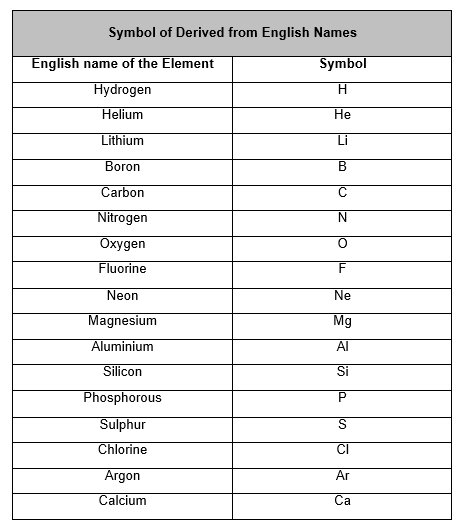Symbols Derived from Latin Names(b) Significance of The Symbol of an Element :

(i) Symbol represents name of the element.

(ii) Symbol represents one atom of the element.

(iii) Symbol also represents one mole of the element. That is, symbol also represent 6.023 × 1023 atoms of the element.

(iv) Symbol represent a definite mass of the element i.e. atomic mass of the element.

Example :

(i) Symbol H represents hydrogen element.

(ii) Symbol H also represents one atom of hydrogen element.

(iii) Symbol H also represents one mole of hydrogen atom.

(iv) Symbol H also represents one gram hydrogen atom.

(c) Atomic Mass of an Element :

Actual masses of the atoms of the elements are very, very small. For example, one atoms of hydrogen (H) has mass of 1.673 × 10-24 gram. To avoid the inconvenience in using such small and complicated figures in our calculation, it was necessary to define atomic mass in such as way that we get simple figures for them. Carbon -12 atom is that atom of carbon which has 6 protons and 6 neutrons in its nucleus, so that its mass number is 12.

Carbon -12 atom has been assigned an atomic mass of exactly 12 atomic mass units, written as 12 u.

Definition of atomic mass : Atomic mass express as to how many time an atom of a substance is heavier than 1/12 mass of the carbon - 12 atom.

Atomic Mass Unit (u)  = One - twelfth the mass of a Carbon - 12 atom or 1u = 1.6605 × 10-24 g.

One atomic mass unit (1u) is defined as exactly one-twelfth the mass of an atom of Carbon-12. The atomic mass of an element is the relative mass of its atom as compared with the mass of a Carbon-12 atom taken as 12 units.

(d) How Do Atoms Exist ?

The atoms of only a few elements called noble gases (such as helium, neon, argon and krypton etc.) are chemically uncreative and exist in the free state (as single atoms). Atoms of most of the elements are chemically very reactive and do not exist in the free state (as single atoms).

Atoms usually exist in two ways :

(i) in the form of molecules and

(ii) in the form of ions.

When atoms form molecules or ins, they become stable (because in doing so they acquire the stable electron arrangements of noble gases).

MOLECULES

A molecule is an electrically neutral group of two (or more) atoms chemically bonded together by means of attractive forces.

OR

A molecule is the smallest particle of a substance (element or compound) which has the properties of that substance and can exist in the free state. Molecules can be formed either by the combination of atoms of the “same element” or of “different elements”/

Every compound is a molecule, but every molecule is not a compound.

There are two types of molecules : molecules of elements and molecules of compounds.

(a) Molecules of Elements :

The molecule of an element contains two (or more) similar atoms chemically bonded together. For example, a molecule of hydrogen element contains 2 hydrogen atoms combined together and it is written as H2 representing Hydrogen gas. Similarly, Ozone gas has 3 oxygen atoms combined together, so ozone exists in the form of O3. The noble gases like helium, neon, argon and krypton etc., exist as single atoms He, Ne, Ar and Kr respectively. So, their atoms and molecules are just the same.

Atomicity of phosphorus is 4 and sulphur is 8.

(b) Molecules of Compounds :

The molecule of compound contains two (or more) different types of atoms chemically bonded together. For example, the molecule of hydrogen chloride (HCI) contains one atom of hydrogen (H) chemically bonded with one atom of chlorine (Cl). Some more example of the molecules of compounds are : sulphur dioxide (SO2), methane, (CH4) and ammonia (NH3).

(c) Atomicity :

The number of atoms present in one molecule of an element or compound is called it atomicity. For example, the atomicity of noble gases is 1, that of hydrogen, nitrogen, oxygen etc. is 2 each and of ozone is 3. Thus, noble gases, hydrogen and ozone are respectively monatomic, diatomic, and triatomic molecules.

A compound which consists of molecules and not ions, is called a molecular compound.

MOLECULAR MASS

The molecular mass of a substance may be defined as the relative mass of its molecule as compared to the mass of an atom of carbon (carbon - 12) taken as 12 units.

Molecular mass expresses as to how many times a molecular of a substance is heavier than 1/12th of the mass of an atom of carbon (carbon -12).

Eg. A molecule of water is 18 times heaver than 1/12th of the mass of carbon atom. Therefore, the molecular mass of water is 18u. Similarly, the molecular mass of CO2 is 44u.

Calculation : The molecular mass is equal to sum of the atomic masses of all the atoms present in one molecule of the substance.

Eg. The molecular mass of Sulphuric Acid (H2SO4) can be calculated as follows :

Molecular mass of H2SO= Mass of 2 H atoms + Mass of 1 S atom + Mass of 4 O atoms

= (2 × 1) + (1 × 32) + (4 × 16) = 2 + 32 + 64 = 94u.

Thus, the molecular mass of Sulphuric acid is 98u.

Atoms are the components of molecules and the molecules are the components of elements or compounds.

IONS

An ion is a positively or negatively charged atom or group of atoms.

Every atom contains equal number of electron (negatively charged) and protons (positively charged). Both charges balance each other, hence atom is electrically neutral.

(a) Cation : If an atom has less electrons than a neutral atom, then it gets positively charged and a positively charged ion is known as cation.

E.g. Sodium ion (Na+), Magnesium ion (Mg2+) etc.

A cation bears that much units of positive charge as are the number of electrons lost by the neutral atom to form that cation.

E.g. An aluminum atom loses 3 electrons to form aluminum ion, so aluminum  ion bears 3 units of positive charge and it is represented as Al3+.

All metal elements form cations.

(b) Anion :If an atom has more number of electrons than that of neutral atom, then it gets negatively charged and a negatively charged iron is known as union.

E.g. Chloride ion (Cl-), oxide ion (O2-) etc.

All non-metal elements form anions (except hydrogen).

An anion bears that much units of negative charge as are the number of electrons gained by the neutral atom to form that anion.

E.g. A nitrogen atom gains 3 electrons to form nitride ion, so nitride ion bears 3 units of negative charge and it is represented as N3-.

Size of a cation is always smaller and anion is always greater than that of the corresponding neutral atom.

MONOATOMIC IONS AND POLYATOMIC IONS

Monoatomic ions : Those ions which are formed form single atoms are called monoatomic ions or simple ions.

E.g.  Na+, Mg2+ etc.

Polyatomic ions : Those ions which are formed from group of atoms joined together are called polyatomic ions or compound ions.

E.g. Ammonium ion ( NH4+), hydroxide ion (OH-) etc. which are formed by the joining of two types of atoms, nitrogen and hydrogen in the first case and oxygen and hydrogen in the second.

IONIC COMPOUNDS

Those compounds which are made up of ions (cations and anions) and are held together by strong electrostatic forces of attraction are called ionic compounds.

The forces which hold the ions together in an ionic compound are known as ionic bonds or electrovalent bonds.

E.g. Calcium nitrate Ca(NO3)2 is an ionic compound, whose one molecule is made up of one calcium ion (Ca2+) and two nitrate ions (NO3), making the overall charge on calcium nitrate zero.

Overall charge on an ionic compound is always zero.

(a) Formula Unit of Ionic Compounds :

The simplest combination of ions that produces and electrically neutral unit,  is called  a formula unit of the ionic compound. Molecular formula of ionic compounds cannot be determined because they consist of large no. of ions. So ionic compounds are represented by formula unit.

Eg. Sodium chloride is an ionic compound which consists of a large number of Na+ and Cl- ions (but they should be equal in number). So, the actual formula of sodium chloride should be (Na+)n(Cl-)n or (Na+Cl-)n, where ‘n’ is a large number. Nacl is the simplest formula of sodium chloride and thus, the formula unit of sodium chloride is NaCl.

FORMULA MASS

The formula mass of an ionic compound is the relative mass of its formula unit as compared with the mass of a carbon atom (carbon - 12) taken as 12 units.

E.g. To find the formula mass of potassium carbonate (K2CO3).

Formula mass of K2CO3 = mass of 2 K atoms + Mass of 1 C atom + Mass of 3 O atoms

= (2 × 39) + (1 × 12) + (3 × 16)

= 78 + 12 + 48

= 138 u .

Hence, the formula mass of K2COis 138 u.

CHEMICAL FORMULA /MOLECULAR FORMULA

The chemical formula of a compound or an element represent the composition of a molecule of the compound or an element, in terms of the symbols of elements and the number of atoms of each element present in one molecule of the substances.

(a) Formulae of Elements :

The chemical formula of an element is a representation of the composition of its molecule in which symbol represents the element and subscript represents, how many atoms are present in one molecule.

E.g. One molecule of hydrogen element contains Two atoms of hydrogen, therefore, the formula of hydrogen is H2. It should, however, be noted that 2H represents two separate atoms of hydrogen, while H2 represents one molecule of hydrogen.

(b) Formulae of Compounds :

The chemical formula of a compound is representation of the composition of its molecule in which symbol represents, which elements are present and the subscript shows us how many atoms of each element are present in one molecule of a compound. E.g. One molecule of water contains 2 atoms of hydrogen and 1 atom of oxygen. Hence, the formula of water is H2O.

(i) In the chemical formula of a compound, the elements present are denoted by their symbols and the number of atoms of each element are denoted by writing their number as subscripts to the symbols of the respective elements.

E.g. Water is a compound whose one molecule is made up of 2 atoms of hydrogen and 1 atom of oxygen and hence, its chemical formula is H2O.

(ii) While writing the formula of an ionic compound, the metal is written on the left hand side, while the non metal is written on the right hand side.

E.g. Magnesium oxide is written as MgO, Sodium chloride is written as NaCl etc.

The name of the metal remains as such, but that of the non-metal is changed to have the ending ‘ide’.

(iii) Molecular compounds, formed by the combination between two different non - metals, are written in such a way that the less electronegative element is written on the left hand side, while the more electronegative element is written on the right hand side. In naming molecular compounds, the name of the less negative non-metal is written as such but the name of the more electronegative element is changed to have the ending ‘ide’.

E.g. H2S is named as hydrogen sulphide and HCI is named as hydrogen chloride.

(iv) When there are more than one atoms of an element present in the formula of the compound, then the number of atoms are indicated by the use of appropriate prefixes (mono for 1, di for 2, tri for 3, tetra for 4 atoms etc. respectively) in the name of the compound.

E.g. CO is named as carbon monoxide, COis named s carbon dioxide and CCI4 is named as carbon tetrachloride.

(v) The prefixes are needed in naming those binary compounds in which the two non-metals form more than one compounds (by having different number of atoms).

E.g. Two non-metals, nitrogen and oxygen, combine to form different compounds like nitrogen monoxide ( NO), nitrogen dioxide (NO2), dinitrogen trioxide (N2O3) etc.

(vi) If two non-metals form only one compound, then prefixes are not used in naming such compounds.

E.g. Hydrogen and sulphur combine to form only one compound H2S. So, H2S is named as hydrogen sulphide and not as hydrogen monosulphide.

In a chemical formula higher electronegative element is written on the right side.

WRITTING THE FORMULA OF MOLECULAR COMPOUND

If we know the valencies of the elements involved in a compound, then we can write the formula of the compound by balancing the valencies of different elements. The steps to be followed for writing the formula of molecular compounds are :

(i) First, write the symbols of the elements constituting the compound.

(ii) Then, below each symbol, write its corresponding valency.

(iii) Finally, we exchange the valencies of the combining atoms, i.e. with first atom, we write the valency of the second atom and second atom, we write the valency of the first atom. The valencies are to be written as subscripts to the symbols.

(iv) If the valencies have any common factor, then the formula is divided by that common factor. This gives the required formula of the compound.

Writing the formula of carbon dioxide as an example : Following steps are used to write the formula of carbon dioxide

Carbon dioxide is a compound composed of two elements, carbon and oxygen. So, we first write their symbols C and O respectively.

The valency of carbon is 4 and the valency of oxygen is 2. Now, these valencies are to be written under the corresponding symbols of elements.Now, the valencies of carbon and oxygen are to be exchanged. So, the subscript corresponding to C is 2 and that corresponding to O is 4. Hence, the formula of the compound becomes C2O4.

But, the valancies 2 and 4 have a common factor 2. So, on dividing the whole formula by 2, we get the simplest formula CO2. Thus, the formula of carbon dioxide is CO2.

VALLENCY OF IONS

The valency of an ion is same as the charge present on the ion.

if an ion has 1 unit of positive charge, its valency is +1 and it is known as a monovalent cation. If an ion has 2 units of negative charge, its valency is -2 and it is known as a divalent anion.

LIST OF COMMON ELECTROVALENT POSITIVE RADICALS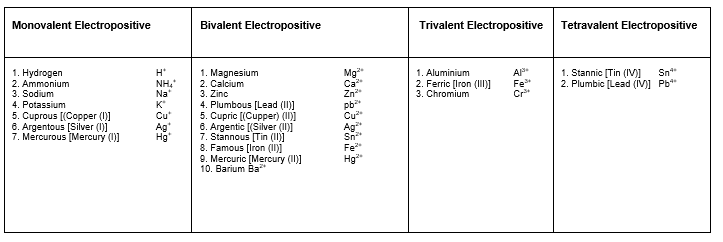LIST OF COMMON ELECTROVALENT NEGATIVE RADICALS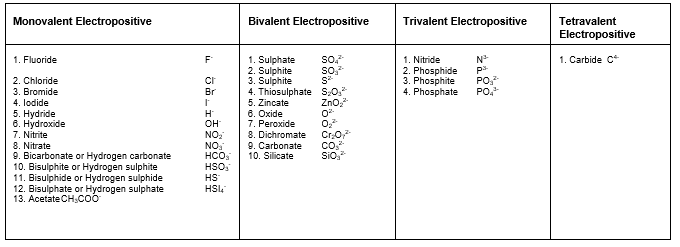Cation contains less no. of electrons and anion contains more no. of electrons than the no. of protons present in them.

WRITING THE FORMULA OF IONIC COMPOUNDS

Steps :

(i) First, write the symbols of the ions from which the ionic compound is made. As a convention, the cation is written on the left side, while the anion is written on the  right side.

(ii) Them the respective valencies of the respective cation and anion are written below their symbols.

(iii) Then, the valencies of the cation and anion are exchanged. The number of cations and anions in the formula of the compound are adjusted in such a way that total positive charge of cations become equal to the total negative charge of the anions making the ionic compound electrically neutral.

(iv) The final formula of the ionic compound is then written but the charges present on the cation and the anion are not shown.

E.g. Two write the formula for aluminum sulphate

First, write the symbols of aluminum ion and sulphate ion and write their respective valencies below their symbols as shown :(Charges)

Now, we exchange the valencies. So, -2 gets associated with Al and +3 gets associated with SO4, So, the final formula of the compound aluminum sulphate is Al2(SO4) after removing the charges associated with aluminum ion and sulpahte ion.

Ionic compound is electrically neutral as it contains equal number of positive and negative charges.

GRAM ATOMIC MASS AND GRAM MOLECULAR MASS

(a) Gram Atomic Mass

The amount of a substance in grams which is numerically equal to the atomic mass of that substance, is known as gram atomic mass of that substance. If we want to write the gram atomic mass of a substance, we write its atomic mass, remove the atomic mass unit u, and add grams to the numerical value of the atomic mass.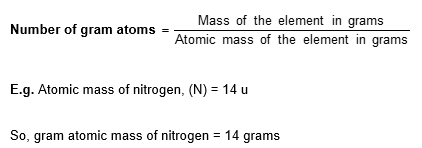(b) Molar Mass :

The molar mass of a substance is the mass of 1 mole, i.e. 6.023 × 1023 particles, of that substance. Its unit is gram per mole, i.e. g/mol. The molar mass of an element is its atomic mass expressed in g/mol and the molar mass of a compound is its molecular mass expressed in g/mol.

E.g. The atomic mass of sodium (Na) is 23 u, so the molar mass of the element sodium (Na) is 23 g/mol.

(c) Gram Molecular Mass:

The amount of a substance in grams which is numerically equal to the molecular mass of that substance, is known as gram molecular mass of that substance. If we want to write the gram molecular mass of a substance, we write its molecular mass, remove the molecular mass unit u, and add grams to the numerical value of the molecular mass.

E.g. gram molecular mass of oxygen gas (O2) is 32 g.Gram molecular mass should not be confused with the mass of one molecule of the substance in grams. The mass of one molecule of a substance is known as its actual mass or molecular mass.

MOLE CONCEPT

For the counting of articles, the unit dozen or unit gross is commonly used irrespective of their nature.

E.g. One dozen pencils        = 12 pencils

One gross books    = 144 books

In a similar way, for counting of atoms, molecules, ions etc. chemists use the unit “mole”.

A mole is the amount of a substance that contains the same number of entities (i.e. atoms, molecules or ions) as there are atoms in 12 grams of the carbon 12 6 C.

Mole i s a collection of 6.023 × 1023 particles (may it be atoms, molecules or ions) of a substance.

E.g. 1 mole of oxygen atoms (O)           = 6.023 × 1023 atoms of oxygen.

1 mole of oxygen molecules (O2)   = 6.023 × 1023 molecules of oxygen.

The number, 6.023 × 1023, which represents the number of particles in a mole, is known as Avogadro Number (NA).

(a) Moles of Atoms :

(i) 1 mole atoms of any element occupy a mass which is equal to the gram atomic mass of that element.

(ii) The symbol of an element represents 6.023 × 1023 atoms (1 mole of atoms) of that element.

E.g. Symbol N represents 1 mole of nitrogen atoms and 2N represents 2 moles of nitrogen atoms.

The terms mole was introduced by Ostwald in 1896.

(b) Moles of Molecules :

(i) 1 mole molecules of any substance occupy a mass which is equal to the gram molecular mass of that substance.

E.g. 1 mole of water (H2O) molecules weight equal to the gram molecular mass of water (H2O), i.e. 18 grams.

(ii) The formula of compound represents 6.023 × 1023 molecules (1 mole of molecules) of that compound.

E.g. Symbol H2O represents 1 more of water molecules and 2 H2O represents 2 moles of water molecules.

NOTE : The symbol H2O does not represent 1 mole of H2 molecules and 1 mole of O atoms. Instead, it represents 2 moles of hydrogen atoms and 1 mole of oxygen atoms.

The SI unit of the amount of a substance is Mole.

(c) Mole in Terms of Volume :

Volume occupied by 1 gram molecular mass or 1 mole of a gas under standard conditions of temperature and pressure (273 K and 1 atm) is called gram molecular volume. Its value is 22.4 litres for each gas.

1 Mole = 1 Gram molecular mass

= 22.4 litre (at NTP)

= 6.023 × 1023 molecules

SOME IMPORTANT RELATIONS REGARDING MOLES OF ATOMS

1. Mass of 1 mole of atoms = Gram atomic mass
2. 1 mole of atoms = 6.023 × 10‑ atoms
3. Gram atomic mass = Mass of 6.023 × 1023 atoms

E.g. to calculate the number of moles in 16 grams of sulphur (Atomic mass of sulphur = 32 u).

Solution :

1 mole of atoms = Gram atomic mass.

So, 1 mole of sulphur atoms = Gram atomic mass of sulphur = 32 grams.

Now, 32 grams of sulphur contains = 1 mole of sulphur

So, 16 grams of sulphur will contain = (1/32) × 16

= 0.5 mole

Thus, 16 grams of sulphur constitute 0.5 mole of sulphur.

Mole is the Latin word meaning heap or pile.

SOME USEFUL FORMULA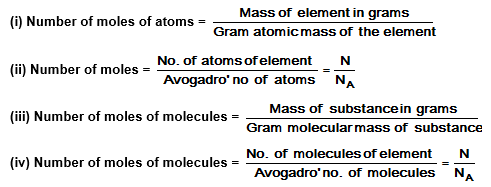SOME USEFUL FORMULA

(i) 1 mole of molecules = Gram molecular mass

(ii) 1 mole of molecules = 6.023 × 1023 molecules

(iii) Gram molecular mass = 6.023 × 1023 molecules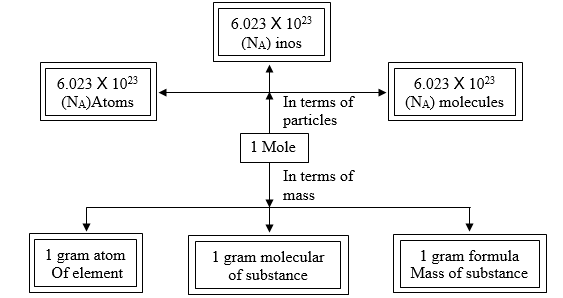SOME USEFUL FORMULAE

Example : Calculate the mass of the following :

(i) 0.5 mole of O2 gas                            (ii) 0.5 mole of O atoms

(iii) 3.011 × 1023 atoms of O                   (iv) 6.023 × 1023 molecules of O2.

(Given : Gram atomic mass of oxygen = 16 g, gram molecular mass of oxygen (O2) = 32 g).

Solution : (i) 0.5 mole of O2 gas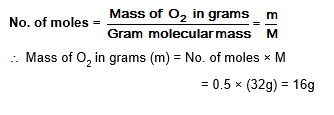Step II : Calculation of mass of oxygen (O) atoms

Mass of oxygen (O) atoms  = Gram atomic mass of oxygen x No. of gram atoms of oxygen.

= 16 × 0.5 = 8g.

(iv) 6.023 × 1023 molecules of oxygen (O2) -

Step I : Calculation of no. of moles of oxygen.

Step II : Calculation of mass of oxygen (O2) molecules.

Mass of oxygen (O2) molecules = Gram molecular mass of oxygen x No. of moles of oxygen.

= 32 × 1 = 32 g

## Tags:

Click for more Science Study Material
 CBSE Class 9 Science Atoms And Molecules Notes Set A CBSE Class 9 Science Atoms And Molecules Notes Set B CBSE Class 9 Science Categories Of Practical Skills Notes CBSE Class 9 Science Diversity In Living Organisms Notes Set A CBSE Class 9 Science Diversity In Living Organisms Notes Set B CBSE Class 9 Science Force And Laws Of Motion Notes Set A CBSE Class 9 Science Force And Laws Of Motion Notes Set B CBSE Class 9 Science Fundamental Unit Of Life Notes CBSE Class 9 Science Gravitation And Fluid Notes Set A CBSE Class 9 Science Gravitation And Fluid Notes Set B CBSE Class 9 Science Gravitation And Fluid Notes Set C CBSE Class 9 Science Improvement In Food Resources Notes Set A CBSE Class 9 Science Improvement In Food Resources Notes Set B CBSE Class 9 Science Is Matter Around Us Pure Notes Set A CBSE Class 9 Science Is Matter Around Us Pure Notes Set B CBSE Class 9 Science List Of Experiments CBSE Class 9 Science Matter In Our Surroundings Notes Set A CBSE Class 9 Science Matter In Our Surroundings Notes Set B CBSE Class 9 Science Motion Notes CBSE Class 9 Science Motion Notes Set A CBSE Class 9 Science Natural Resources Notes Set A CBSE Class 9 Science Natural Resources Notes Set B CBSE Class 9 Science Physical Science Notes CBSE Class 9 Science Revision Notes CBSE Class 9 Science Sound Notes CBSE Class 9 Science Structure Of Atom Notes Set A CBSE Class 9 Science Structure Of Atom Notes Set B CBSE Class 9 Science The Fundamental Unit Of Life Notes CBSE Class 9 Science Tissue Notes Set A CBSE Class 9 Science Tissue Notes Set B CBSE Class 9 Science Wave Motion And Sound Notes CBSE Class 9 Science Why Do We Fall Ill Notes Set A CBSE Class 9 Science Why Do We Fall Ill Notes Set B CBSE Class 9 Science Work Energy And Power Notes Set A CBSE Class 9 Science Work Energy And Power Notes Set B

## Latest NCERT & CBSE News

Read the latest news and announcements from NCERT and CBSE below. Important updates relating to your studies which will help you to keep yourself updated with latest happenings in school level education. Keep yourself updated with all latest news and also read articles from teachers which will help you to improve your studies, increase motivation level and promote faster learning

### Moderation of Marks Class 11 and 12 Board Exams

The portal for moderation and finalization of results for Class-12 is being opened from 16.07.2021 to 22.07.2021. As Board has to declare the result latest by 31.07.2021, schools have been requested to follow the schedule strictly and complete the moderation within...

This has a reference to the letter no CBSE/COORD/2021 dated 11.02.2021 regarding conduct of the School-Based Assessment (Internal Assessment/Practical/ Project) of Class-XII and uploading the marks by 11.06.2021. It has been observed that some schools have not been...

### CBSE Board Examination Scheme of Assessment 2021 2022

A. Academic session to be divided into 2 Terms with approximately 50% syllabus in each term: The syllabus for the Academic session 2021-22 will be divided into 2 terms by following a systematic approach by looking into the interconnectivity of concepts and topics by...

### FAQs on Policy for Tabulation of Marks for Class 10 Board Exams

ON POLICY Question.1 How CBSE will declare the result of Class-X? Answer. Results of Class X Board will be declared on the basis of an objective criterion developed by the Board vide Notification no.CBSE/CE/2021 dated 01.05.2021. Question.2. If any candidate is not...

### CBSE Class 12 Evaluation Criteria 2021

SUMMARY OF THE POLICY FOR TABULATION OF MARKS FOR CLASS XII BOARD EXAMINATIONS 2021   1(a) Due to cancellation of the Board examinations, the assessment of theory portion of 80/70/60/50/30 marks will be done by the school based on the following:     Class...

×